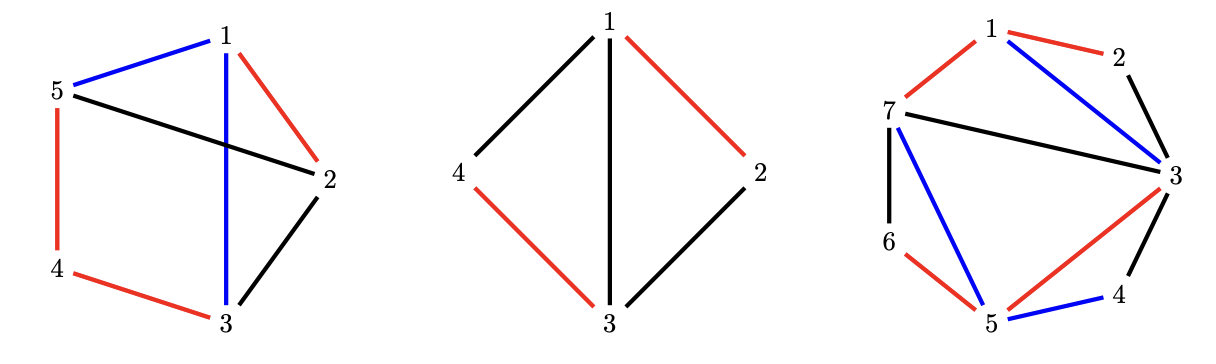시간 제한 메모리 제한 제출 정답 맞은 사람 정답 비율
3 초 512 MB 2 2 2 100.000%

## 문제

“...fool me once, shame on — shame on you. Fool me — you can’t get fooled again.” – W.

In this task, we will observe regular polygons that have each of their N sides colored in one of three colors and whose vertices are denoted from 1 to N in a clockwise order. A triangulaton of a polygon is a decomposition of its area into a set of non-overlapping triangles whose sides either lie on the sides of the polygon or its internal diagonals. Of course, in this task, each of the diagonals used for polygon triangulation is also colored in one of three colors.

The triangulation is said to be patriotic if each of its N − 2 triangles has all three sides of different colors. Your task is to determine whether a given polygon with its diagonals is triangulated and whether that triangulation is patriotic.

## 입력

The frist line contains the number of subtask this particular test case belongs to (see the table in the scoring section). If your solution doesn’t care about that, simply read the number and feel free to ignore it.

The second line contains an integer N from the task description.

The third line contains an integer consisting of N digits which represent the colors of polygon sides. More precisely, the first digit represents the color of side (1, 2), the second digit represents the color of side (2, 3), and so on until the N-th digit which represents the color of side (N, 1). The colors will always be denoted with digits 1, 2 and 3.

Each of the next N − 3 lines contain a description of one diagonal in the form X Y C, where X and Y are polygon vertices and C is the color of the diagonal (1 ≤ X, Y ≤ N, 1 ≤ C ≤ 3). Each line describes a valid diagonal, i.e., vertices X and Y will neither be equal nor neighbouring.

## 출력

If the input polygon is not correctly triangulated, you should output "neispravna triangulacija" (invalid triangulation in Croatian).

If the input polygon is correctly triangulated but the triangulation is not patriotic, you should output "neispravno bojenje" (invalid coloring in Croatian).

If the input polygon is correctly triangulated and that triangulation is patriotic, you should output "tocno" (correct in Croatian).

## 제한

• 4 ≤ N ≤ 2 · 105

## 예제 입력 1

1
5
12113
1 3 3
2 5 2


## 예제 출력 1

neispravna triangulacija


## 예제 입력 2

1
4
1212
1 3 2


## 예제 출력 2

neispravno bojenje


## 예제 입력 3

1
7
1223121
1 3 3
3 5 1
5 7 3
7 3 2


## 예제 출력 3

tocno


## 힌트

Clarifications of examples: